# GMAT Math : Understanding decimals

## Example Questions

### Example Question #1 : Decimals

Rewrite as a fraction in lowest terms: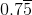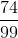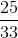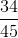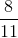Explanation: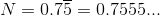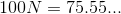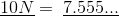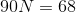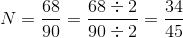### Example Question #1 : Understanding Decimals

Add one hundred seven ten-thousandths to seventeeen one-hundredths.

Two hundred seventy-seven one-thousandths

One thousand one hundred seventy-seven ten-thousandths

One hundred eighty-seven one-thousandths

One thousand eight hundred seven ten-thousandths

One thousand seven hundred seventeen ten-thousandths

One thousand eight hundred seven ten-thousandths

Explanation:

One hundred seven ten-thousandths = 0.0107

Seventeeen one-hundredths = 0.17, or 0.1700

The sum: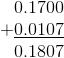This is one thousand eight hundred seven ten-thousandths.

### Example Question #3 : Decimals

Multiply seventy-seven one-hundredths by sixty-six one-thousandths.

Five hundred eighty-two ten-thousandths

Five thousand eighty-two hundred-thousandths

Five thousand eighty-two ten-thousandths

Forty-six thousand six hundred sixty-two hundred-thousandths

Five hundred eighty-two hundred-thousandths

Five thousand eighty-two hundred-thousandths

Explanation:

Seventy-seven one-hundredths = 0.77

Sixty-six one-thousandths = 0.066

The product: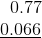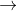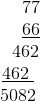0.77 and 0.066 have a total of five digits to the right of their decimal points, so position the decimal point in the answer so that there are five digits to its right.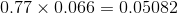This is five thousand eighty-two hundred-thousandths.

### Example Question #4 : Decimals

Divide eight hundred eighty-eight thousandths by sixty-four ten-thousandths.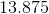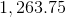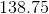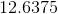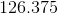Explanation:

Eight hundred eighty-eight thousandths = 0.888

Sixty-four ten-thousandths = 0.0064

Set up a long division: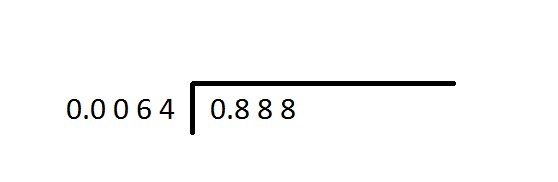Move the decimal point four places right in both numbers so that the divisor will be a whole number. Note that this will require the use of placeholder zeroes in the dividend.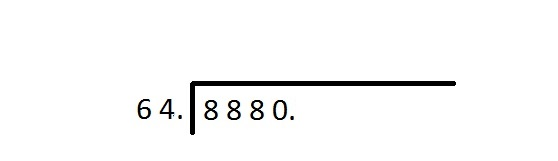(Note that leading zeroes have been removed.)

Carry out the long division, making sure you align the decimals: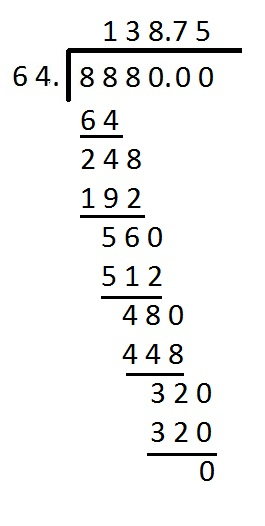The quotient of this division is that of the original problem, so the correct choice is 138.75.

### Example Question #5 : Decimals

Which of the following is the square of twenty seven one-thousandths?

Seven hundred twenty-nine one-hundred-thousandths

The correct answer is not among the other responses.

Fifty-four one-millionths

Seven hundred twenty-nine one-millionths

Fifty-four one-hundred-thousandths

Seven hundred twenty-nine one-millionths

Explanation:

Twenty-seven one-thousandths = 0.027

Its square is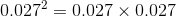Multiply without regard to the decimals first: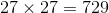0.027 and 0.027 have a total of six digits to the right of their decimal points, so position the decimal point in the answer so that there are six digits to its right.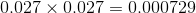This is seven hundred twenty-nine one-millionths.

### Example Question #6 : Decimals

Which of the following is the cube of fifteen one-hundredths?

The correct answer is not among the other responses.

Three thousand three hundred seventy-five one-millionths

Forty-five one-millionths

Forty-five one-hundred-thousandths

Three thousand three hundred seventy-five one-hundred-thousandths

Three thousand three hundred seventy-five one-millionths

Explanation:

Fifteen one-hundredths = 0.15

Its cube is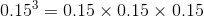Multiply without regard to the decimals first: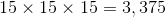0.15, 0.15, and 0.15 have a total of six digits to the right of their decimal points, so position the decimal point in the answer so that there are six digits to its right.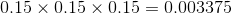This is three thousand three hundred seventy-five one-millionths.

### Example Question #7 : Decimals

Subtract nine hundred six ten-thousandths from four tenths.

Three thousand ninety-four ten-thousandths

Three hundred ninety-four thousandths

Three hundred ninety-four ten-thousandths

Five hundred six thousandths

Three thousand nine hundred four ten-thousandths

Three thousand ninety-four ten-thousandths

Explanation:

Nine hundred six ten-thousandths = 0.0906

Four tenths = 0.4, or 0.4000

The difference: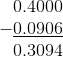This is three thousand ninety-four ten-thousandths.

### Example Question #8 : Decimals

Which of these equations is not correct?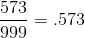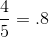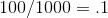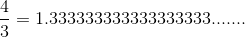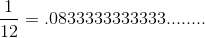Explanation:

Although tempting, a calculator is not required to spot the correct answer.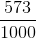is equal to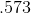as it is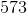parts of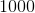. And therefore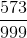must be slightly different. (It's it actually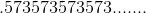)

### Example Question #9 : Decimals

If we divide 10 by 3, what is the 10th digit after the decimal point of the quotient?Explanation: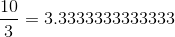The tenth digit after the decimal point is 3.

When dividing 1 by 3 or 10 by 3, we get repeating 3s. So, there must be a 3 in the tenths digit.

### Example Question #10 : Decimals

Ifandare positive integers such that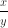= 4.46, which of the following numbers could be the remainder whenis divided by?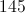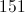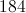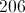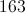Explanation:

Given that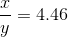it follows that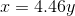.

We don't need to knowor, the only important information is that: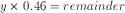Where the remainder is any one of the answer choices. This is because the remainder for any divisor/dividend pair is the product of the decimal portion of the quotient and the divisor.

Since we don't know y, our answer choice for the remainder is any answer that satisfies the above equation for a positive interger, sinceis specified as such in the problem.

The only answer choice that satisfies the constraints onis 184: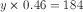==>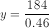==>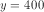Tired of practice problems?

Try live online GMAT prep today.